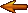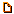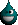# Topic: Calculus Anyone? (Page 1 of 1)SleepingWolf
Paranoid (IV) Inmate

From:
Insane since: Jul 2006posted 09-15-2008 20:00

Limit as b approaches t

b^4-t^4
---------
b^5-t^5

I remember the derivative for a quotient, but much too rusty to solve this one.

Nature & Travel Photography
Main Entrance

Arthurio
Paranoid (IV) Inmate

From: cell 3736
Insane since: Jul 2003posted 09-15-2008 22:02

I trust my old friend Mathcad with things like these :PSleepingWolf
Paranoid (IV) Inmate

From:
Insane since: Jul 2006posted 09-15-2008 22:38
quote:

Arthurio said:

I trust my old friend Mathcad with things like these :P

Thanks...that is the correct answer..but how do you get to it??

Nature & Travel Photography
Main Entrance

Arthurio
Paranoid (IV) Inmate

From: cell 3736
Insane since: Jul 2003posted 09-15-2008 23:35

No idea ^^

SleepingWolf
Paranoid (IV) Inmate

From:
Insane since: Jul 2006posted 09-15-2008 23:53

Ok ..thanks again.
I'm going to try to divide one function into the other.

Nature & Travel Photography
Main Entrance

hyperbole
Paranoid (IV) Inmate

Insane since: Aug 2000posted 09-16-2008 04:07

Let

code:
`t = b + h`

then solve for lim(h->0).

.

-- not necessarily stoned... just beautiful.SleepingWolf
Paranoid (IV) Inmate

From:
Insane since: Jul 2006posted 09-16-2008 04:24
quote:

hyperbole said:

Let code:t = b + h then solve for lim(h->0)..-- not necessarily stoned... just beautiful.

Sorry, but you lost me there..why introduce h?

Nature & Travel Photography
Main Entrance

Slime

From: Massachusetts, USA
Insane since: Mar 2000posted 09-16-2008 05:36

L'hopital's rule. Differentiate the numerator and the denominator with respect to b:

numerator becomes 4*b^3
denominator becomes 5*b^4

The result is ((4*b^3) / (5*b^4)) = (4 / (5 * b))

Then it's safe to just plug in b = t and get 4/(5*t).SleepingWolf
Paranoid (IV) Inmate

From:
Insane since: Jul 2006posted 09-16-2008 05:40
quote:

Slime said:

L'hopital's rule. Differentiate the numerator and the denominator with respect to b:numerator becomes 4*b^3denominator becomes 5*b^4The result is ((4*b^3) / (5*b^4)) = (4 / (5 * b))Then it's safe to just plug in b = t and get 4/(5*t).

Thanks Slime. My mistake was differentiating both b and t - at the same time.
It' s been a long time ..Much appreciated.

Nature & Travel Photography
Main Entrance

hyperbole
Paranoid (IV) Inmate

Insane since: Aug 2000posted 09-16-2008 05:55
code:
```lim     { b^4 - t^4 }
b-->t   ------------
{ b^5 - t^5 }

let t = b+h

lim     { b^4 - (b+h)^4 }
h-->0   ----------------
{ b^5 - (b+h)^5 }

lim     { b^4 - (b^4 + 4b^3h + 6b^2h^2 + 4bh^3 + h^4) }
=  h-->0   -------------------------------------------------------------------
{ b^5 - (b^5 + 5b^4h + 10b^3h^2 + 10b^2h^3 + 5bh^4 + h^5) }

lim     { -4b^3h - 6b^2h^2 - 4bh^3 - h^4 }
=  h-->0   -------------------------------------------------
{ -5b^4h - 10b^3h^2 - 10b^2h^3 - 5bh^4 - h^5 }

lim     { -h(4b^3 + 6b^2h + 4bh^1 + h^3) }
=  h-->0   ---------------------------------------------------
{ -h(5b^4 + 10b^3h + 10b^2h^2 + 5bh^3 + h^4) }

lim     { 4b^3 + 6b^2h + 4bh^1 + h^3 }
=  h-->0   ----------------------------------------------
{ 5b^4 + 10b^3h + 10b^2h^2 + 5bh^3 + h^4 }

as h goes to 0 all the terms wtih h drop out leaving

4b^3
=   ----
5b^4

4
=   --
5b```

.

-- not necessarily stoned... just beautiful.(Edited by hyperbole on 09-16-2008 05:59)

SleepingWolf
Paranoid (IV) Inmate

From:
Insane since: Jul 2006posted 09-16-2008 13:52

Hyperbole:

Very interesting...thanks for taking the time to walk through that one.

Given that all expressions with h drop to 0, you're right back with the derivatives of the original functions.
Is this meant to be the longer proof?

Could this also have been solved with the quotient rule?

Nature & Travel Photography
Main Entrance

wrayal
Bipolar (III) Inmate

From: Cranleigh, Surrey, England
Insane since: May 2003posted 09-16-2008 19:44

Hyperbole's derivation is an approach from first principles - this is the actual definition of the limit, and where all differentiation comes from. E.g. for the general polynomial x^n:

d/dx(x^n)=lim(h->0)( (x+h)^n - x^n )/h (with appropriate assumptions about convergence)

= lim(h->0)(x^n + hnx^n-1 + ... + h^n - x^n)/h
= lim(h->0)( nhx^n-1 + O(h^2) )/h
= nx^n-1.

The product rule is a similar idea:

lim(h->0) (f(x+h)g(x+h))-(f(x)g(x))/h
= lim(h->0) (f(x+h)g(x+h)-f(x+h)g(x)+f(x+h)g(x)-f(x)g(x))/h (since -f(x+h)g(x)+f(x+h)g(x)=0)
= lim(h->0) f(x+h)g'(x) + g(x)f'(x)
= f(x)g'(x)+g(x)f'(x)

(again, with sufficient conditions on existence of differentials)

However, the quotient rule shows why the conditions are necessary: it's very tempting to simply sub in g(x)=k(x)^-1 for some k(x) and use the chain rule to produce the answer. This works in the majority of cases, but requires extra rigour to handle the exceptional cases - in practice, a slightly more fiddly proof is used for the quotient rule.

(analysis is fun ^_^)SleepingWolf
Paranoid (IV) Inmate

From:
Insane since: Jul 2006posted 09-17-2008 04:53

Thanks!

Nature & Travel Photography
Main Entrance

hyperbole
Paranoid (IV) Inmate

Insane since: Aug 2000posted 09-17-2008 17:55

When I first read your problem. with it being stated as a limit, I thought about solving the limit. I didn't think about it as a dervative until Slime pointed out the use of L'Hopital's rule.

As wrayal said, all derivatives are derived from the function

code:
`F'(x) = lim(h-->0) (F(x+h) - F(x)) / h`

I remember reading, many years ago when I was a Freshman, that Newton never used the rules we use today for solving derivatives. He always used limits to solve his calculus problems. The rules such as F'(x^n) = nx^(n-1) were found later and were derived from the limit function I showed above. I'd hate to have to go through Physics using limits to solve problems.

.

-- not necessarily stoned... just beautiful.wrayal
Bipolar (III) Inmate

From: Cranleigh, Surrey, England
Insane since: May 2003posted 09-22-2008 02:17

Hehe, if you think that's a pain, consider this: Newton produced his laws of planetary motion without calculusTo be accurate, he solved them at first using calculus (as anyone in their right mind would today) but wasn't confident in his new tool, so in fact produced geometric proofs for every theorem! As an example, consider the following theorem:

A particle at distance l from the centre of a planet of uniform density, radius r where r>l (notice the direction of the inequality) will be affected by gravity in the same way as if it were simply sitting on the surface of a planet of the same uniform density radius l. That is, all matter of the planet between the shells radius l and r can be neglected for the calculation of its instantaneous motion. Now *that* is easier by calculus, and yet if you check his Principia...